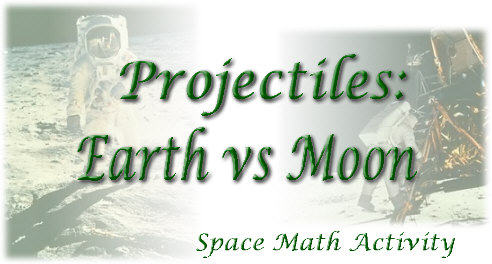NASA Project NOVA Activity Developer:  Donna Roberts

Activity:  To determine and examine the path taken by a bottle cap projectile on the earth and on the moon.

Mathematical Skills:  grade level 9-12, graphing and solving quadratic equations, graph interpretation, using a graphing utility (a TI-84+ graphing calculator was used here)

Materials:  graph paper, graphing calculator

Directions:

Earth: On Earth, it is possible to shoot a bottle cap 64 feet straight up into the air with a rubber band.  In t seconds after firing, the bottle cap is

s(t) = 64t - 16t2 feet above your hand.
 a.) Draw a graph that simulates the position of the bottle cap on earth. b.) How long does it take the bottle cap to reach its maximum height? c.) What is the maximum height reached by the bottle cap?

Moon: On the moon, the same force will send the bottle cap to a height of
s(t) = 64t - 2.6t2
feet in t seconds.
 a.) Draw a graph that simulates the position of the bottle cap on the moon. b.) Approximately, how long does it take the bottle cap to reach its maximum height? c.) Estimate the maximum height reached by the bottle cap to the nearest tenth of a foot?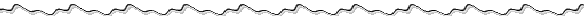For the teacher:

While this problem can be solved with paper and pencil, the use of the graphing calculator will offer students a visual interpretation of the problem and reinforce graphing concepts.

One possible solution using the TI-84+ graphing calculator: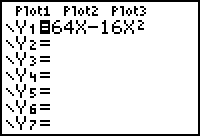Type the equation into Y1. Discuss why using the variable  "x" will yield the same result as using the variable "t".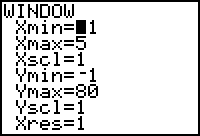Determine a window by trial and error or by a prior algebraic solution obtained by setting the function equal to zero and solving for t.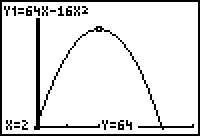Hit graph.   Is this value seen in the window after hitting "trace"  the actual maximum value?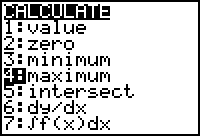Find the actual maximum value using the calculator's maximum function. (found  under "calc" above the "trace" key)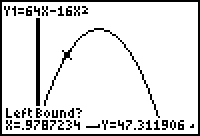The maximum function will ask for a left bound.  Move the "spider"  (cursor) to the left of where you  imagine the maximum to be located. Hit enter.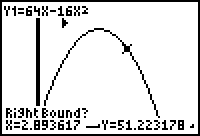Move the "spider" to the right of where you imagine the maximum to be located. Hit enter.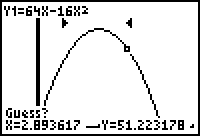When asked to "guess", just press "enter."  Notice the pointers surrounding the area where the maximum will occur.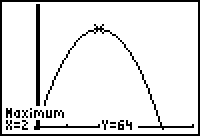Hitting "enter" will yield the  x and y values of the maximum.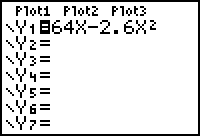Repeat the process for the  second equation.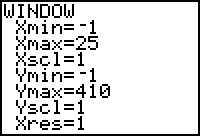Possible window.  Students should predict if the time span will increase and discuss why or why not.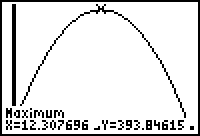Answer. Students should discuss why the same force created different paths for the bottle cap on the earth and on the moon.  This is a good opportunity to engage in "writing in the mathematics classroom."